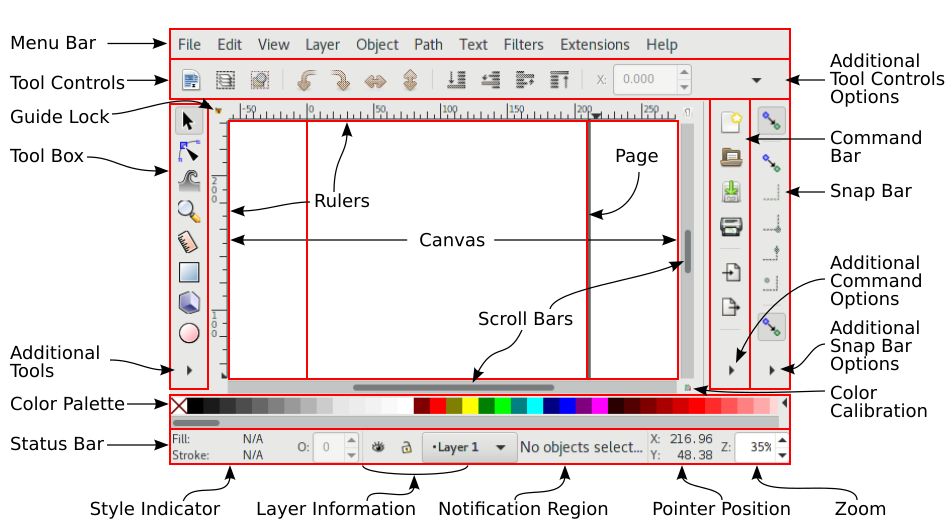# SVG - Bezier Curve

A path can be describe as a series of Bezier curves

There are an infinite number of Bezier curves, but only two simple ones are available in path elements:

• a cubic one, called with C,
• and a quadratic one, called with Q.

## Type

### C - Cubic Bezier Curve Command

To create a smooth curve, we need two more points, the control points. They describe the slope of the curve at the start and end point.

• C x1 y1, x2 y2, x y
• c dx1 dy1, dx2 dy2, dx dy

where:

• x,y is where the line end
• x1,y1 is the control point for the start point (where the path is)
• x2,y2 is the control point for the end point (for the point x,y)

The control points describe:

• the slope of your line starting at each point.
• and the force

The curve starts in the direction of the first control point, and then bends so that it arrives along the direction of the second control point.

Below you have the same line but with two different control point vector.

``````<svg width="190" height="160" xmlns="http://www.w3.org/2000/svg">

<path d="M 10 10 C 10 20, 110 20, 110 10" />
<line x1=10 y1=10 x2=10 y2=20 />
<line x1=110 y1=10 x2=110 y2=20 />

<path d="M 10 60 C 10 100, 110 100, 110 60" />
<line x1=10 y1=60 x2=10 y2=100 />
<line x1=110 y1=60 x2=110 y2=100 />

</svg>```
```
``````path {
stroke:black;
fill:transparent;
}
line {
stroke:red;
}```
```

### S - Cubic Poly Bezier Curve Command

S will string together several Bezier curves to create extended, smooth shapes.

• S x2 y2, x y
• s dx2 dy2, dx dy

where:

• x2, y2 is the control point of the end point
• x,y is the end point
• The control point of the first point is computed automatically as the reflection of the control point for the previous “C” command relative to the start point of the “S” command.
• If the S command doesn't follow another S or C command, then it is assumed that both control points for the curve are the same.

Example:

``````<!-- Without S -->
<svg width="190" height="160" xmlns="http://www.w3.org/2000/svg">
<path d="M 10 80 C 40 10, 65 10, 95 80" stroke="black" fill="none" />
<!-- The line vector created by the control point -->
<line x1=10 y1=80 x2=40 y2=10 stroke="red"/>
<line x1=95 y1=80 x2=65 y2=10 stroke="red" />
</svg>
<!-- The same with S -->
<svg width="190" height="160" xmlns="http://www.w3.org/2000/svg">
<path d="M 10 80 C 40 10, 65 10, 95 80  S 150 150, 180 80" stroke="black" fill="transparent"/>
<!-- The line vector created by the control point -->
<line x1=10 y1=80 x2=40 y2=10 stroke="red"/>
<line x1=95 y1=80 x2=65 y2=10 stroke="red" />
<!-- the reflection of the control point 65 10 with as center the end point of C  95 80 -->
<line x1=95 y1=80 x2=125 y2=150 stroke="red" />
<line x1=150 y1=150 x2=180 y2=80 stroke="red" />
</svg>
<!-- Without C -->
<svg width="190" height="160" xmlns="http://www.w3.org/2000/svg">
<path d="M 10 80 S 150 150, 180 80" stroke="black" fill="transparent"/>
<!-- The line vector created by the control point -->
<line x1=10 y1=80 x2=150 y2=150 stroke="red"/>
<line x1=150 y1=150 x2=180 y2=80 stroke="red" />
</svg>```
```

To continue

## Documentation / Reference

Recommended PagesInkscape - Bezier Mode

Bezier in InkscapeSVG - Curve (Line)

in SVG. A curved line is just designed with a path element. There are three different path commands that you can use to create smooth curves. bezier curve, an “arc” or part of a circle....